# 4. (a) Assume a function h is differentiable at some point to. Is it true that...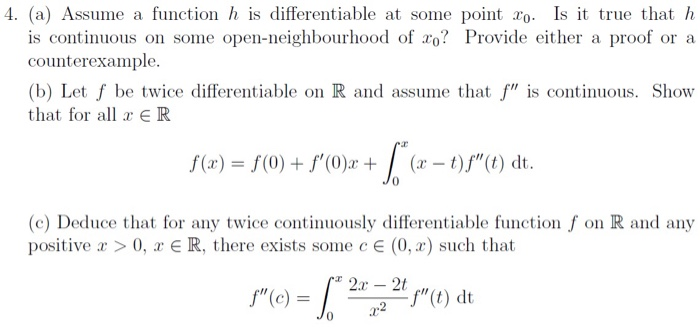4. (a) Assume a function h is differentiable at some point to. Is it true that h is continuous on some open-neighbourhood of xo? Provide either a proof or a counterexample. (b) Let f be twice differentiable on R and assume that f" is continuous. Show that for all x ER S(x) = S(0) + s°C)x + [ (x - 1))"(dt. (C) Deduce that for any twice continuously differentiable function f on R and any positive x > 0, x € R, there exists some c € (0, 2) such that 2.r - 2t .22 -f"(t) dt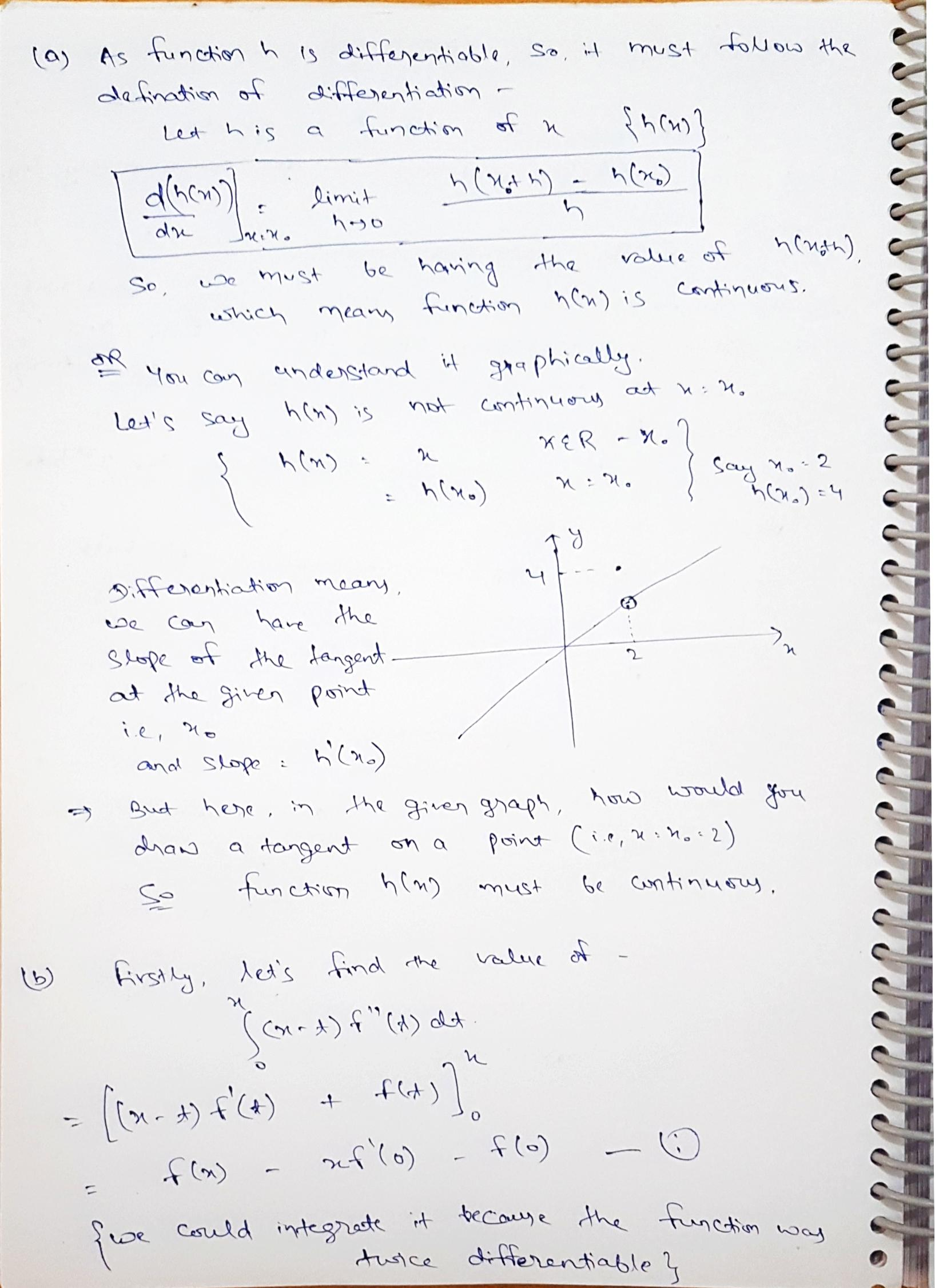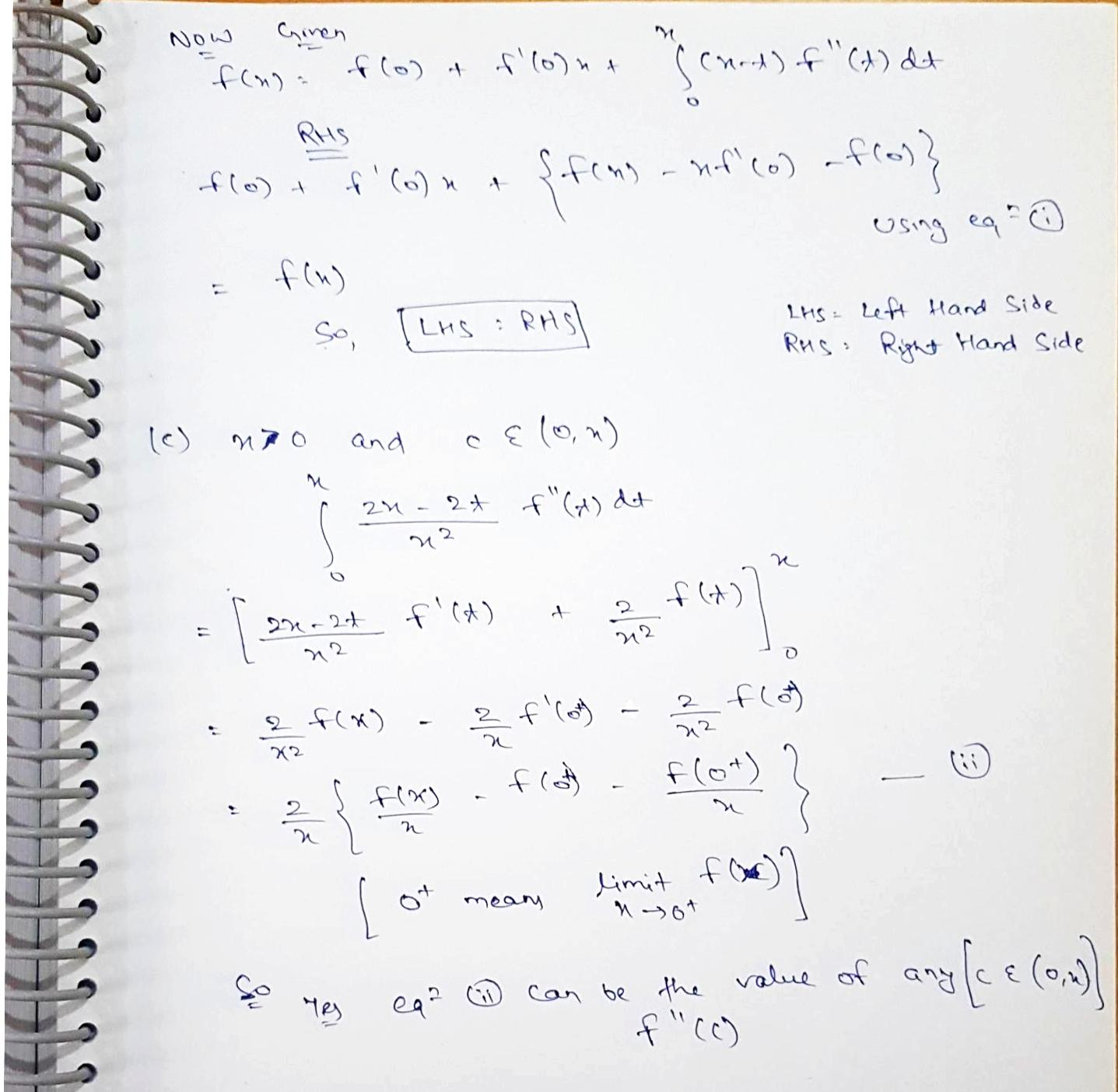##### Add Answer of: 4. (a) Assume a function h is differentiable at some point to. Is it true that...
Similar Homework Help Questions
• ### 3. Let f: R+R be a function. (a) Assume that f is Riemann integrable on [a,...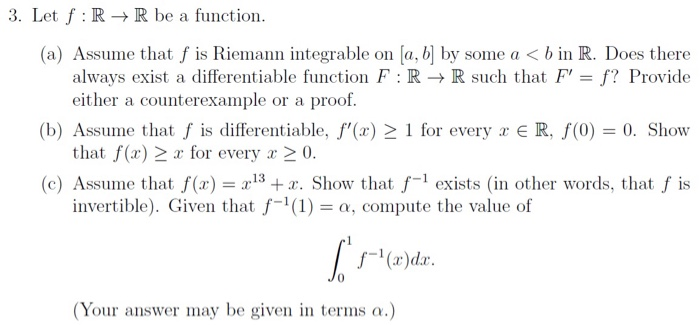3. Let f: R+R be a function. (a) Assume that f is Riemann integrable on [a, b] by some a < b in R. Does there always exist a differentiable function F:RR such that F' = f? Provide either a counterexample or a proof. (b) Assume that f is differentiable, f'(x) > 1 for every x ER, f(0) = 0. Show that f(x) > x for every x > 0. (c) Assume that f(x) = 2:13 + x. Show that...

• ### Assume f : R" → R is twice continuously differentiable. Prove that the following are equivalent: ...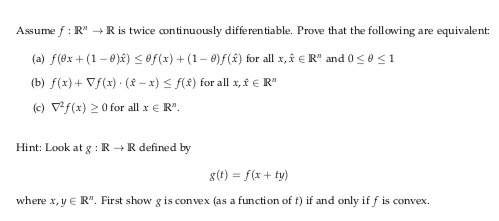Assume f : R" → R is twice continuously differentiable. Prove that the following are equivalent: (a) f(ex + (1-8)ì) < ef(x) + (1-8)/(x) for all x, x E Rn and 0 < θ < 1 (b) f(x)+ /f(x) . (x-x) -f(r) for all x,x E R" (c) f(x) > 0 for all x E R" Hint: Look at : RRdefine by gt) f(x + ty) where x, y E R. First show g is convex (as a function of...

• ### V:RR is continuously differentiable. Define the Suppose that the function function g : R2R by 8(s...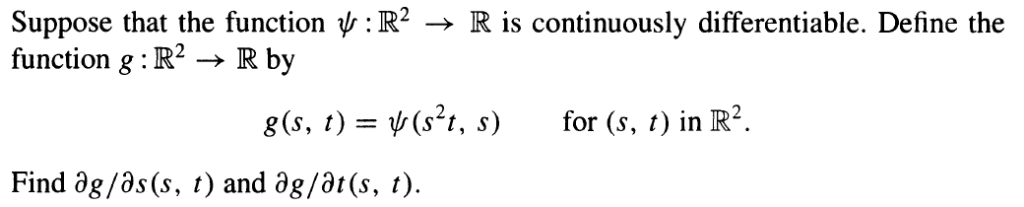Please prove by setting up the theorem below (Chain Rule) v:RR is continuously differentiable. Define the Suppose that the function function g : R2R by 8(s, t)(s2t, s) for (s, t in R2. Find ag/as(s, t) and ag/at(s, t) Theorem 15.34 The Chain Rule Let O be an open subset of R and suppose that the mapping F:OR is continuously differentiable. Suppose also thatU is an open subset of Rm and that the functiong:u-R is continuously differentiable. Finally, suppose that...

• ### Let f be a real-valued continuous function on R with f (-o0 0. Prove that if f(xo) > 0 for some z...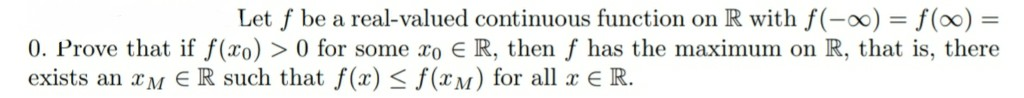Let f be a real-valued continuous function on R with f (-o0 0. Prove that if f(xo) > 0 for some zo R, then f has the maximum on R, that is, there exists an M R such that f(x) < f(xM) for al E R. Let f be a real-valued continuous function on R with f (-o0 0. Prove that if f(xo) > 0 for some zo R, then f has the maximum on R, that is, there exists...

• ### Differentiable FunctionsSuppose that g(x) is differentiable on (0,1) and g'(x)1 for any x (0,1). Show that if g(a)=a andg(b)=b for some a,b (0,1) then a=b.I dont know where to start with this.So by Rolle's Theorem: Let f : [a,b] becontinuous on [a,b] and differentiable on (a,b). If f(a)=f(b), then there exists a point c (a,b) where f'(c)=0. The proof forthis says that because f is continuous on a compact set, f attains a maximum and a minimum. If both the maximum...

• ### 4. Let F be a continuously differentiable function, and let s be a fixed point of...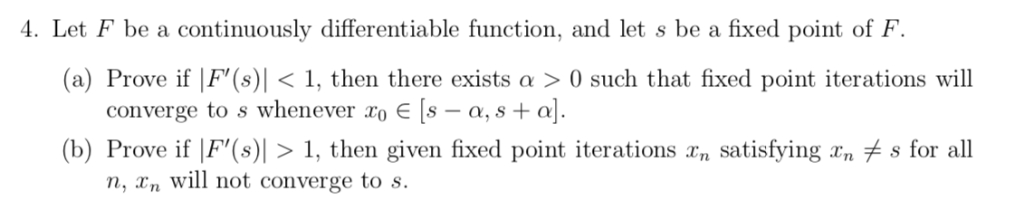4. Let F be a continuously differentiable function, and let s be a fixed point of F (a) Prove if F,(s)| < 1, then there exists α > 0 such that fixed point iterations will o E [s - a, s+a]. converge tO s whenever x (b) Prove if IF'(s)| > 1, then given fixed point iterations xn satisfying rnメs for all n, xn will not converge to s.

• ### Let U be an open subset of R. Let f: U C Rn → Rm. (a) Prove that f is continuously differentiable if and only if for ea...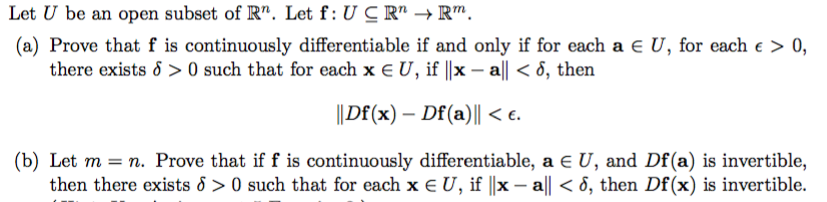Let U be an open subset of R. Let f: U C Rn → Rm. (a) Prove that f is continuously differentiable if and only if for each a є U, for each E > 0, there exists δ > 0 such that for each x E U, if IIx-all < δ, then llDf(x)-Df(a) ll < ε. (b) Let m n. Prove that if f is continuously differentiable, a E U, and Df (a) is invertible, then there exists δ...

• ### real analysis 1,2,3,4,8please 5.1.5a Thus iff: I→R is differentiable on n E N. is differentiable on / with g&#39...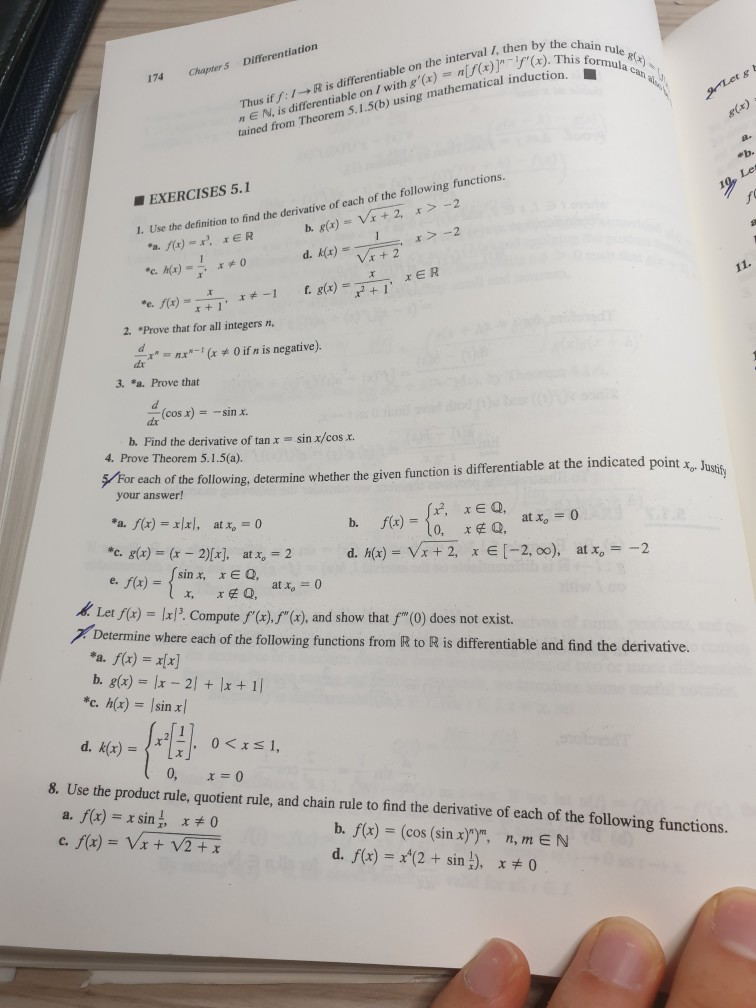real analysis 1,2,3,4,8please 5.1.5a Thus iff: I→R is differentiable on n E N. is differentiable on / with g'(e) ()ain tained from Theorem 5.1.5(b) using mathematical induction, TOu the interal 1i then by the cho 174 Chapter s Differentiation ■ EXERCISES 5.1 the definition to find the derivative of each of the following functions. I. Use r+ 1 2. "Prove that for all integers n, O if n is negative). 3. "a. Prove that (cosx)--sinx. -- b. Find the derivative...

• ### 5. (This question shows an alternative definition for the term differentiable, one that is useful when...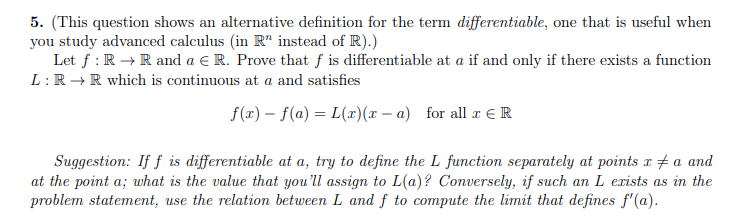5. (This question shows an alternative definition for the term differentiable, one that is useful when you study advanced calculus (in R" instead of R).) Let f: R+R and a ER. Prove that f is differentiable at a if and only if there exists a function L:RR which is continuous at a and satisfies f(x) - f(a) = L(x)(x - a) for all x ER Suggestion: If f is differentiable at a, try to define the L function separately at...

• ### Question 4. For S: B(ro, 0), assume that f: S R" is a function such that...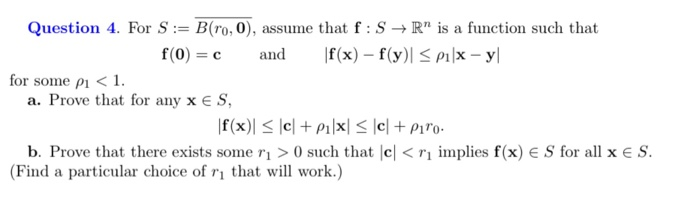Question 4. For S: B(ro, 0), assume that f: S R" is a function such that f(x) f(y)Plx - y f(0) c and for some pi1 a. Prove that for any x E S f(x) elpilx|< \cl + Pi*o b. Prove that there exists some rı > 0 such that c|< r1 implies f(x) e S for all x E S (Find a particular choice of ri that will work.) Question 4. For S: B(ro, 0), assume that f: S...

Free Homework App